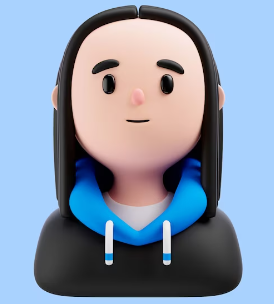#####GET QUOTE IN 2 MINUTES*(5/5)

### block of memory contains 50 random words of data. Assume that the values are in two’s complement representations and are stored in the R/W memory area.

INSTRUCTIONS TO CANDIDATES

Question 1

A block of memory contains 50 random words of data. Assume that the values are in two’s complement representations and are stored in the R/W memory area. You need to provide the 50 random words and to include them in an initialization file: assign2.ini. Write a program using the ARM assembly programming language to do the following:

1. a) You are required to reverse the word order in a block of 50 random words (Hint: the last word stored in the memory becomes the first and vice versa).
2. b) Next, find the minimum and maximum value in the 50 random words of data.

Question 2

1. a) Write a subroutine using the ARM assembly programming language to construct tables of 20 (n = 0, 1, 2, …, 19) 32-bit data. There are 2 inputs to the subroutine. One is an option and the other is the starting address of the table to be constructed. There are 2 possible options: 1 or 2. This option will dictate how you should construct the table. This is shown as followed:

option = 1: Build a table of an arithmetic sequence numbers. This sequence has a difference of 3 between each number. The pattern is continued by adding 3 to the last number each time, like this: 1, 4, 7, 10, 13, 16, 19, 22, 25, ... Assume that the first number is 1.

option = 2: Build a table of a geometric sequence numbers. This sequence has a factor of 3 between each number. The pattern is continued by multiplying by 3 to the last number, like this: 1, 3, 9, 27, 81, 243, ... Assume that the first number is 1.

1. b) Write the main program using the ARM assembly programming language to call the subroutine written in part (2a). The main program needs to provide the 2 inputs to the subroutine: an option and the starting address of the table to be constructed.

Hint: you need to use the key concepts of table generation, jump table, and subroutine.

(5/5)

## Related Questions

##### . Introgramming & Unix Fall 2018, CRN 44882, Oakland University Homework Assignment 6 - Using Arrays and Functions in C

DescriptionIn this final assignment, the students will demonstrate their ability to apply two ma

##### . The standard path finding involves finding the (shortest) path from an origin to a destination, typically on a map. This is an

Path finding involves finding a path from A to B. Typically we want the path to have certain properties,such as being the shortest or to avoid going t

##### . Develop a program to emulate a purchase transaction at a retail store. This program will have two classes, a LineItem class and a Transaction class. The LineItem class will represent an individual

Develop a program to emulate a purchase transaction at a retail store. Thisprogram will have two classes, a LineItem class and a Transaction class. Th

##### . SeaPort Project series For this set of projects for the course, we wish to simulate some of the aspects of a number of Sea Ports. Here are the classes and their instance variables we wish to define:

1 Project 1 Introduction - the SeaPort Project series For this set of projects for the course, we wish to simulate some of the aspects of a number of

##### . Project 2 Introduction - the SeaPort Project series For this set of projects for the course, we wish to simulate some of the aspects of a number of Sea Ports. Here are the classes and their instance variables we wish to define:

1 Project 2 Introduction - the SeaPort Project series For this set of projects for the course, we wish to simulate some of the aspects of a number of

### Ask This Question To Be Solved By Our ExpertsGet A+ Grade Solution Guaranteed##### Lovedeep SinghComputer science
(/5)

Hire Me##### Onkar LapateComputer science
(/5)

Hire Me